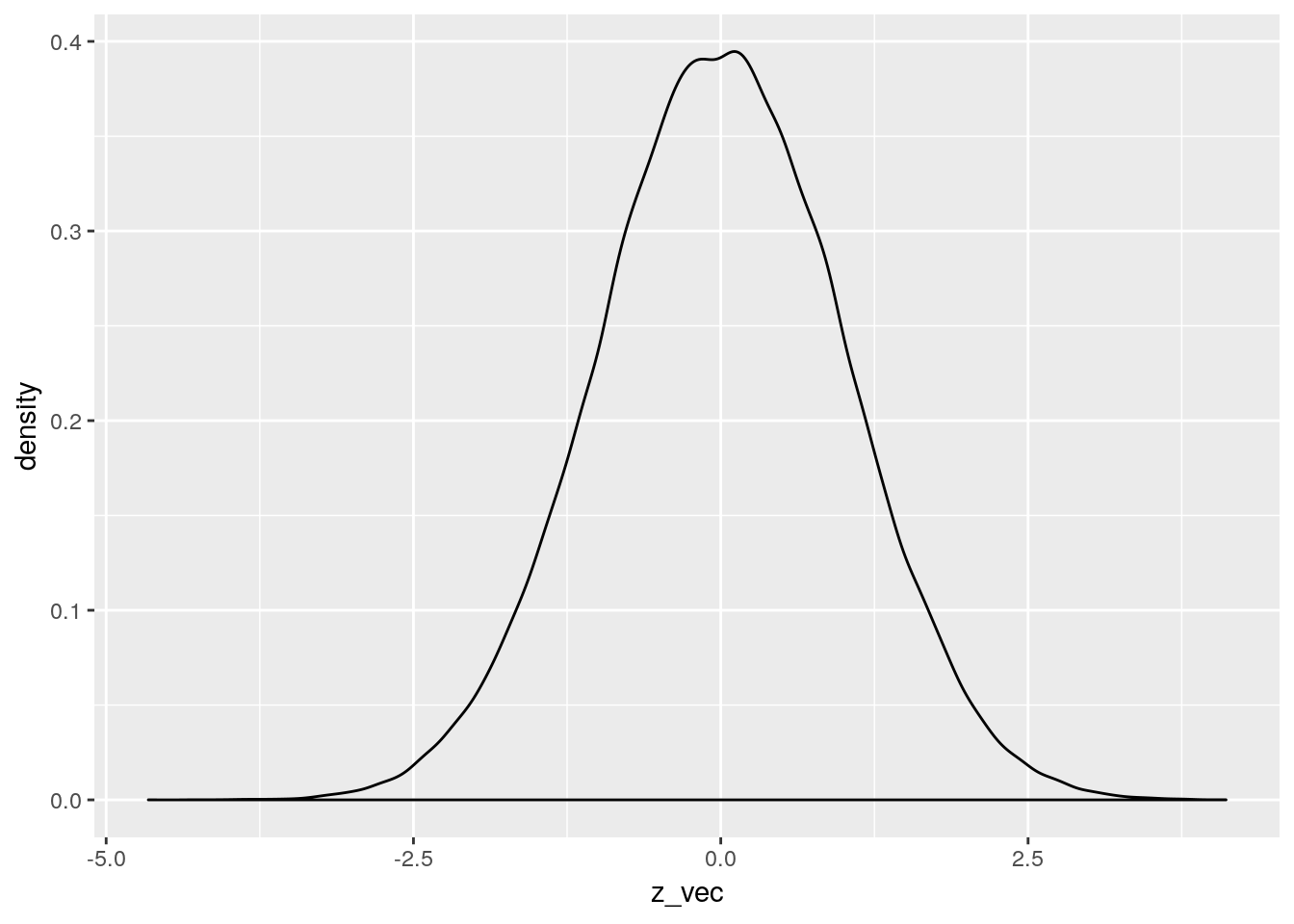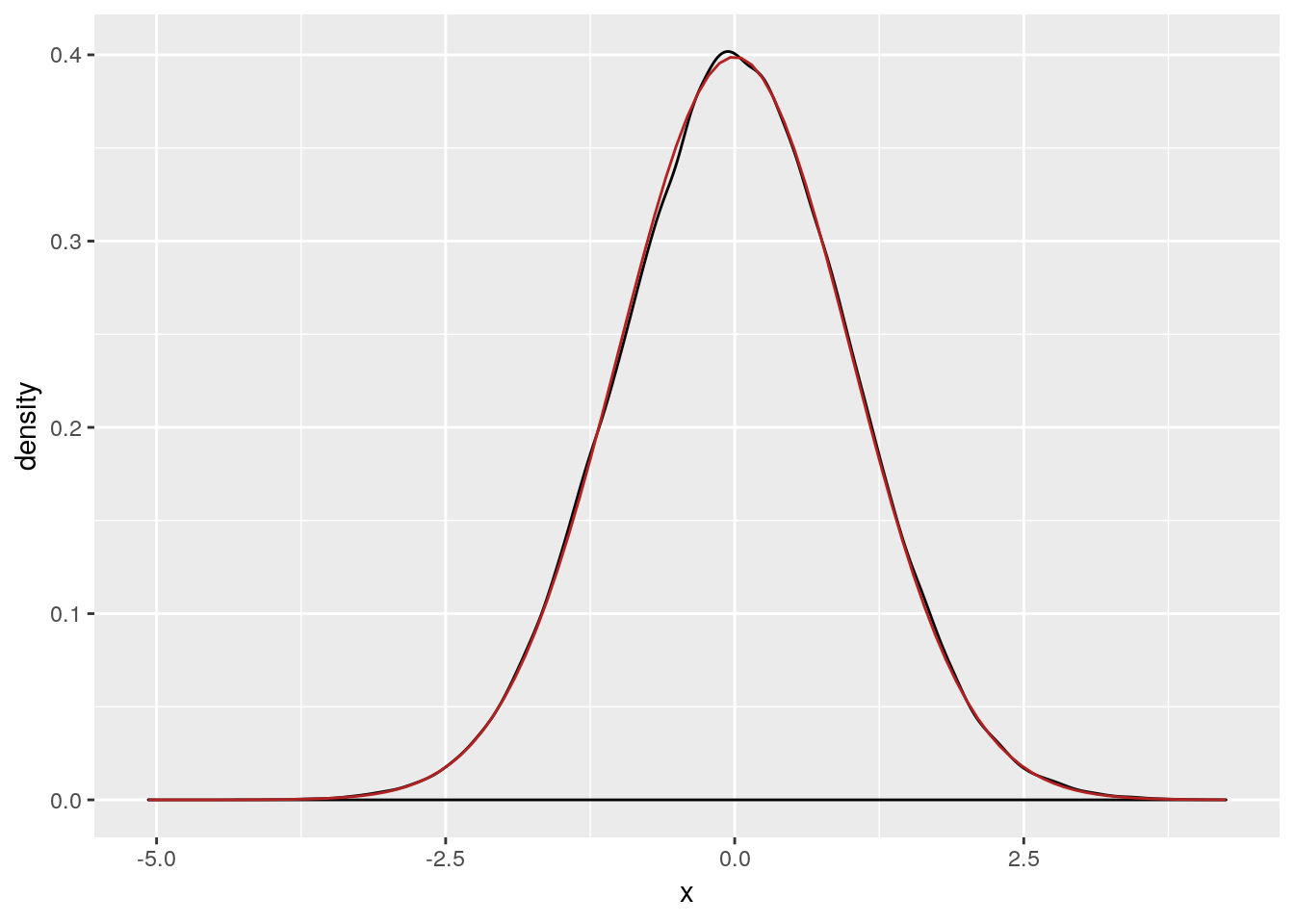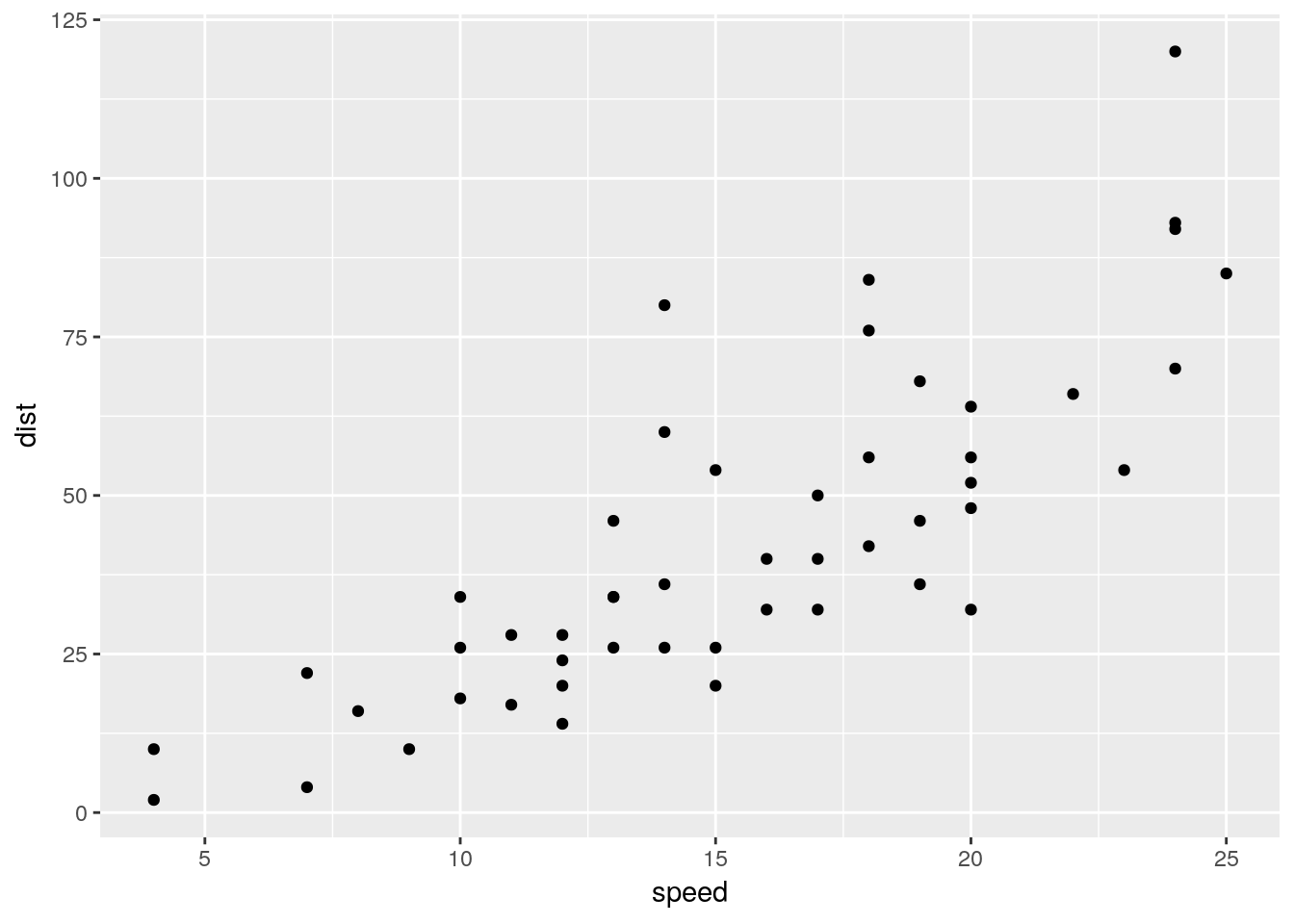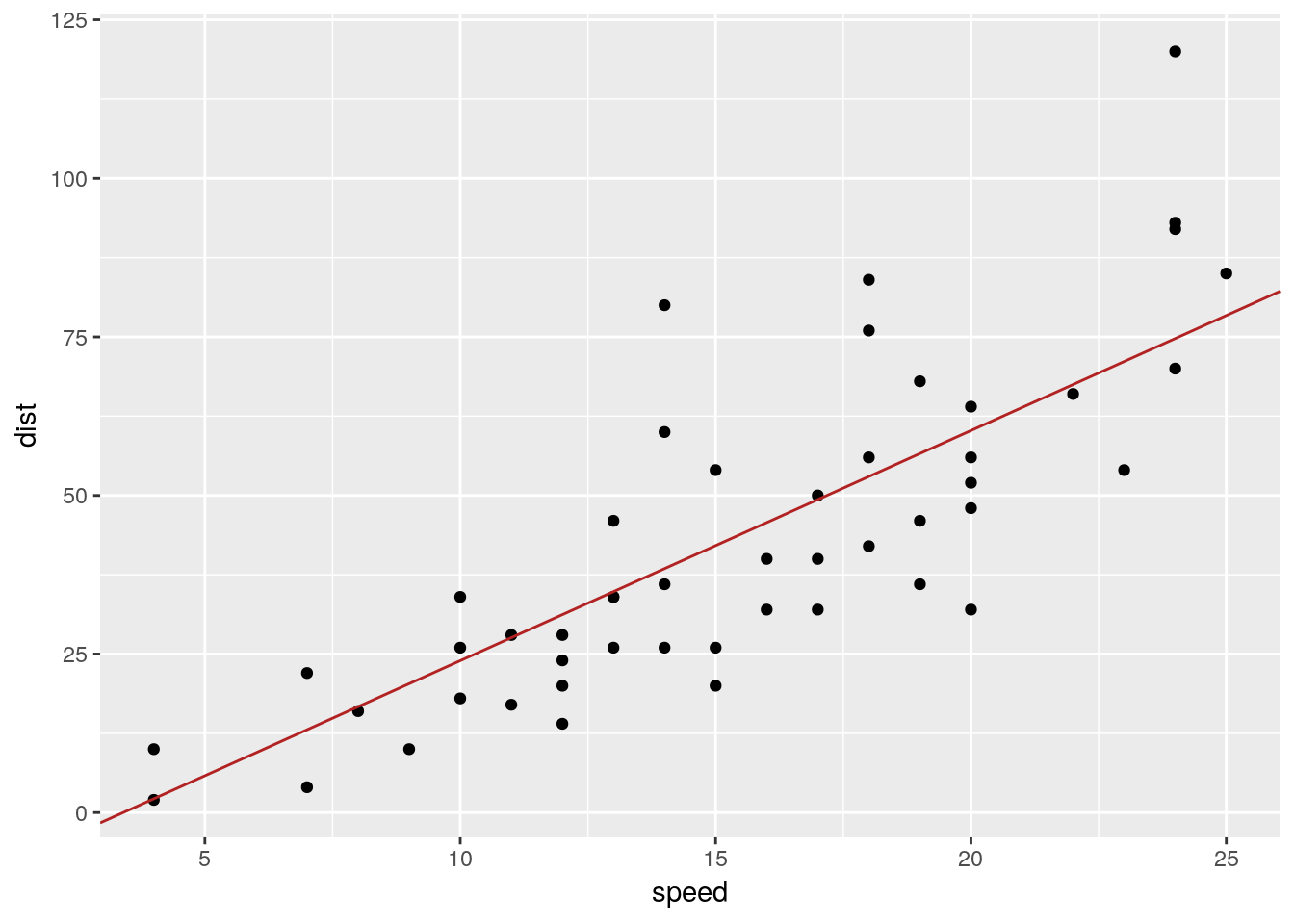# Instructions

• Download and extract this zip file. You will find the R Markdown (.Rmd) file inside.
• Open the .Rmd file in RStudio.
• Fill in the required code and answers.
• ‘Knit’ the document (ctrl/cmd + shift + K in RStudio) to produce a HTML file.
library(tidyverse)
library(TeachingDemos)

char2seed("bayes")

Let’s start by fixing the seed, so that we can compare the outcome of random executions as we go along.

# 1. Simulating the sampling distribution of a $$z$$-test

The $$z$$-test relies on a sampling distribution captured by random variable:

$Z = \frac{\bar{X} - \mu_0}{\sigma / \sqrt{n}}$

It is possible to prove that $$Z$$ follows a standard normal distribution. It is also possible to use numerical simulations to “validate” this claim. (Obviously, this is NOT the same as a mathematical proof!) To do so, we assume that $$H_0$$ is true, say that the random variable $$X$$ follows a normal distribution with $$\mu_0 = 100$$. We also assume that $$\sigma$$ is known, say $$\sigma = 15$$. We then repeatedly sample $$n$$ values (let’s fix $$n = 25$$) from $$X$$. For each sample, we calculate the test statistic. Suppose we do all this $$k$$ times and then plot the data as a density plot. The outcome should (under increasing $$k$$) look more and more like a standard normal distribution. – Let’s do this in R!

## a. Get a vector of values for the test statistic

We start by creating a vector of values for the test statistic. We could use a for loop, but we can also use a more compact, functional style and use map_dbl.

k <- 5    # number of hypothetical experiments
n <- 25     # number of samples to draw in each hypothetical experiment
mu_0 <- 100
sigma <- 15

z_vec = map_dbl(1:k, function(i) {
x_vec = rnorm(  FILL ME )
z = FILL ME
return(z)
})

The solution should be:

z_vec
##  -0.8238208  1.3909726 -0.4984277  0.6411338  0.3960600

## b. Plotting the result

Rerun the code from above, but now take a much larger number of hypothetical experiments, say $$k = 100,000$$. Create a density plot, using geom_density() from package ggplot2.

# # first run the code from the last part with k=100,000
#
z_plot = tibble(z_vec) %>% ggplot( FILL ME ) + FILL ME
z_plot

The outcome should look something like this:## c. Add density curve for standard normal distribution to the plot

Now let’s add a density curve for the standard normal distribution. You can either do this by filling in the template below. Alternatively use stat_function() for a more elegant solution.

z_plot + geom_line(data = tibble(x= seq( FILL ME ), y = dnorm(x, mean = FILL ME, sd = FILL ME)),
aes(x = x, y = y),
color = "firebrick")

The outcome should look something like this:# 2. Linear regression example

Let’s consider the built-in data set cars from R, which contains information about the speed a car was traveling and the distance it required to come to a halt. (Find out more about it by typing help('cars')).

cars = as.tibble(cars)
cars
## # A tibble: 50 x 2
##    speed  dist
##    <dbl> <dbl>
##  1     4     2
##  2     4    10
##  3     7     4
##  4     7    22
##  5     8    16
##  6     9    10
##  7    10    18
##  8    10    26
##  9    10    34
## 10    11    17
## # ... with 40 more rows
1. Plot the data

Make a scatter plot of the cars data with speed on the $$x$$ axis and distance on the $$y$$ axis:

FILL ME

Your outcome should look somegthing like this:Use the hand-made maximum likelihood estimation function from Tuesday’s class and apply it to the case at hand.

## code from previous class

nll = function(y, x, beta_0, beta_1, sd) {
if (sd <= 0) {return( Inf )}
yPred = beta_0 + x * beta_1
nll = -dnorm(y, mean=yPred, sd=sd, log = T)
sum(nll)
}
fit_lh = optim(par = c(0, 1, 1),
fn = function(par) with(murder_data,
nll(murder_rate, low_income,
par, par, par)))
fit_lh\$par

##  -12.331594   3.628442  15.425966

Look back at the code, make sure you understand what it does and that you understand what these numbers mean!

1. Plot the regression line from your results

Use the function geom_abline to also draw the regression line that corresponds to your results from the previous part.

FILL ME + geom_abline(intercept = FILL ME,
slope = FILL ME,
color = "firebrick")## d. Built-in linear regression

Compute a linear regression model with R’s built-in function lm and show the summary() of the output.

lm( FILL ME,  data = cars )

This should look like this:

##
## Call:
## lm(formula = dist ~ speed, data = cars)
##
## Residuals:
##     Min      1Q  Median      3Q     Max
## -29.069  -9.525  -2.272   9.215  43.201
##
## Coefficients:
##             Estimate Std. Error t value Pr(>|t|)
## (Intercept) -17.5791     6.7584  -2.601   0.0123 *
## speed         3.9324     0.4155   9.464 1.49e-12 ***
## ---
## Signif. codes:  0 '***' 0.001 '**' 0.01 '*' 0.05 '.' 0.1 ' ' 1
##
## Residual standard error: 15.38 on 48 degrees of freedom
## Multiple R-squared:  0.6511, Adjusted R-squared:  0.6438
## F-statistic: 89.57 on 1 and 48 DF,  p-value: 1.49e-12

How would you interpret the $$p$$-values in the output? How would you verbalize these results in a research paper?

## e. Expert question

Compare the estimates for the coefficients in the output of lm with those obtained with the hand-made MLE function. What’s going on? Can you find the mistake?